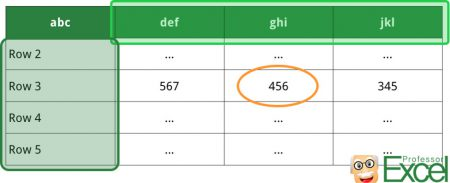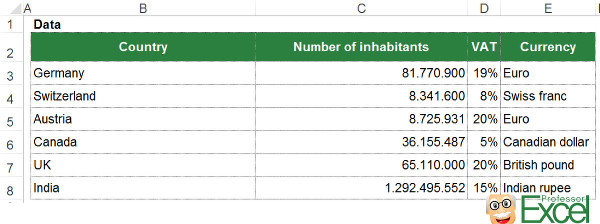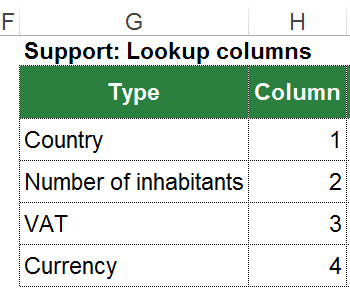# 2D Lookups in Excel: 4 Easy Methods and Examples!

There are many cases in which you need a 2 dimensional lookup. That means, if you want to get a value from a specific row-column combination with neither rows or columns fixed. Unfortunately, the problem of a two way lookup comes up quite often. In this article we explore 4 methods of how to conduct 2D lookups in Excel.

+++NEW+++NEW+++NEW+++ 2D XLOOKUP +++NEW+++NEW+++NEW+++

## In a hurry?

Most common for 2D lookups are INDEX/MATCH/MATCH (see method number 4 below) or a 2D XLOOKUP (click here for more information).

## What is a 2D lookup?At 2D lookups, you search for the row and column at the same time.

In many cases, you only look for a value in – let’s say – column A and return the corresponding value from column B. But there are also many examples, in which you don’t know the return column. For example, depending on the specifications, you want to return the value from either column B, C or D and so on. In such case, we are speaking of 2D lookups or a two way lookup. So we search in both rows and columns at the same time.

## Preparations: The basic formulas for 2D lookups

The following methods are based on 4 basic formulas in Excel. Each formula we’ve described before, so please refer to these articles:

## The four methodsExample: Our table contains 3 data types for each country: The number of inhibitants, the VAT and the currency.

Before we jump in with the four methods, let’s take a look at our example first.

You got the simple table as shown in the image. In has data for different countries. For each country there are 3 types of data: The number of inhabitants, the VAT as well as the currency.

The task: Depending on the country and the data type you want to get the correct value from the table. For example, you select “Canada” and “VAT”, you want to get 5% in return.

Below this table, we want to be able to define the country in cell C11 (here: “Canada”) and the data type in cell C12 (here: “VAT”). Depending on the combination, we want to retrieve the correct value from the data table above.

### Method 1: The easiest way (VLOOKUP & MATCH)

Probably the easiest – or at least the shortest – way of returning values in 2 search dimensions is the VLOOKUP and MATCH combination. We start by setting up a normal VLOOKUP. But instead of setting a fixed value for the return column we use the MATCH formula finding the return column.

The formula in our example looks like this:

`=VLOOKUP(C11,B2:E8,MATCH(C12,B2:E2,0))`

The MATCH formula searches for the type, e.g. VAT, within the range B2 to E2. It returns the number of cell, in which it first comes up.

Closely related is a slightly different version: HLOOKUP with the MATCH formula. The HLOOKUP searches for “VAT” in the first row (row 2) and the match defines the number of the return row. =HLOOKUP(C12,B2:E8,MATCH(C11,B2:B8,0))

### Method 2: You want to stay with VLOOKUP? (VLOOKUP & VLOOKUP)Support table with column numbers for the VLOOKUP formula.

You are not familiar with the MATCH formula and just want to stay with VLOOKUP, you could go with this solution. We start the same way as in method one: We prepare the VLOOKUP formula for searching for the country name in column B. Instead of the return column number (“col_index_num”) we insert another VLOOKUP.

At this step, we need one more preparation. We set up a lookup table with the titles of your data. The second column (column H in the image) contains the number of column starting with 1 for the first title (in our case the country). The second VLOOKUP searches within this table and returns the number of columns.

The complete formula for the 2D Lookup looks like this:

`=VLOOKUP(C11,B2:E8,VLOOKUP(C12,G:H,2,FALSE),FALSE)`

### Method 3: The inconvenient way (INDEX & INDIRECT & VLOOKUP & MATCH)

The third method is not really convenient as it is quite long. We’ve added it here is it shows an approach using the INDIRECT formula. You can transfer it – if you like – to the SUMIFS formula.

We need a bit more preparation for this method. We extend the support table from our method 2 above by the column letter (e.g. VAT = D:D). In order to keep it simple, we don’t simply write D but D:D as this is the complete return range.

In case of the VAT, the normal INDEX/MATCH approach without a dynamic search column would look like this: =INDEX(D:D,MATCH(C11,B:B;0))

With the MATCH formula we search for “Canada” in column B. It returns the number of the first occurrence of “Canada” within this range.

Now – in order to make also the return column a variable – you insert the INDIRECT formula and replace the “D:D”:

`=INDEX(INDIRECT(VLOOKUP(C12,G2:I6,3,FALSE)),MATCH(C11,B:B,0))`

That way, you search within the support table for “VAT”. Once found, it returns “D:D”. With the INDIRECT formula you really make it a part of the INDEX formula and can refer to the range “D:D”.

Do you want to boost your productivity in Excel?

Get the Professor Excel ribbon!

Add more than 120 great features to Excel!

### Method 4: The elegant way for 2D lookups: INDEX / MATCH / MATCH

This is probably the most elegant and most popular way for 2D lookups in Excel: Using the INDEX/MATCH/MATCH combination. Instead of just a simple INDEX/MATCH combination, you can add one more dimension with the second MATCH. The formula looks like this:

` =INDEX(B2:E8,MATCH(C11,B2:B8,0),MATCH(C12,B2:E2,0))`

The INDEX formula has 3 parts here: The complete cell range (B2 to E8) and two MATCH formulas.

1. The return cell range stretches over the hole table. In our case that’s B2 to E8.
2. The first MATCH searches within the range B2 to B8 for the country.
3. The second MATCH looks for the type in the cell range B2 to E3 (the headline).

Both MATCH formulas return the number of the cell in with the search term the first time comes up.

### Method 5 (new): 2D XLOOKUP

Excel now has a new function – XLOOKUP. It’s offers a great alternative to the INDEX/MATCH/MATCH 2D lookup. Check it out here.

## Bonus: How to change from percentage to normal number?The cell is formatted as % when VAT is selected – otherwise “normal” number.Use a conditional formatting rule for changing the %-sign when you display the VAT.

You’ve probably noticed, that in our example for the 2D lookups, the format of the return cell changes depending on the type of value. For instance, when you retrieve the value for VAT, the return cell is formatted with the %-sign. For other values, it shows normal number formats.

1. First step: Set the format of cell C13 to “Number”. In our case, we also use thousand separators. Text is still shown as text so we already got the value types “Number of inhabitants” and “Currency” covered.
2. We only have to set up the exception for “VAT”. This can be done with conditional formatting. Click on “Conditional Formatting” in the center of the Home ribbon. Then click on “New Rule”.
3. In the lower dropdown list, choose “Use a formula to determine which cells to format”.
4. The condition is quite straightforward: =\$C\$12=”VAT”.
5. Define the format if your condition is true. In our case: Number format “Percentage”.
6. Click “OK”.

Please note: Changing the number format with conditional formatting only works with Excel for Windows. As of now, this option is missing in the Mac version.Henrik Schiffner is a freelance business consultant and software developer. He lives and works in Hamburg, Germany. Besides being an Excel enthusiast he loves photography and sports.

1.Shahid Sial says:

very useful article

1.Henrik Schiffner says:

Thank you!

2.Ingrid says:

Is there a way to combine SUMIF or SUMIFS to this to allow for multiple entries. For example if Canada had two rows?

3.Nikunj Parasrampuria says:

Very educating and useful article. In-depth and detailed explanation from every aspect where a person is most likely to make a mistake. Helped me big time. Thanks.

PS: It worked for me after pressing CTRL + SHIFT + ENTER, didn’t work with just ENTER, a necessary step for the formula it seems. If it helps I used the Method 4. Maybe this information should be a part of the article as well, I maybe wrong though. Did anyone go through the issue as me?